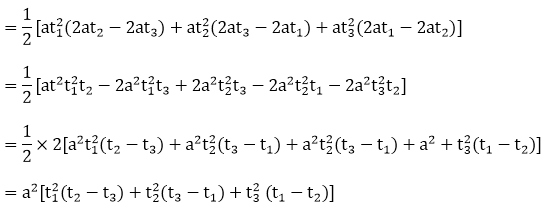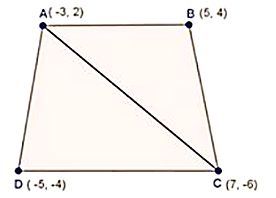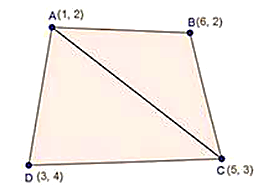#### Thank you for registering.

One of our academic counsellors will contact you within 1 working day.

Please check your email for login details.

Click to Chat

1800-1023-196

+91-120-4616500

CART 0

• 0
MY CART (5)

Use Coupon: CART20 and get 20% off on all online Study Material

ITEM
DETAILS
MRP
DISCOUNT
FINAL PRICE
Total Price: Rs.

There are no items in this cart.
Continue Shopping• Complete JEE Main/Advanced Course and Test Series
• Offered Price: Rs. 15,900
• View Details

# Chapter 14: Coordinate Geometry Exercise – 14.5

### Question: 1

Find the area of a triangles whose vertices are

(i) (6, 3), (-3, 5) and (4, - 2)

(ii) [(at12, at1),( at22, 2at2)( at32, 2at3)]

(iii) (a, c + a), (a, c) and (-a, c - a)

### Solution:

(i) Area of a triangle is given by

1/2[x1(y2 - y3) + x2(y3 - y1) + x3(y1 + y2)]

Here, x1 = 6,y1 = 3, x2 = -3, y2 = 5, x3 = 4,y3 = -2]

Let A(6, 3), B(-3, 5) and C(4,-2) be the given points

Area of ∆ABC = 1/2 [6(5+2)+(-3)(- 2 -3)+ 4(3 - 5)]

=1/2 [6 × 7- 3 × ( – 5) + 4( – 2)]

= 1/2[42 +15 - 8]

= 49/2 sq. units

(ii) Let A = (x1, y­1) = (at12, 2at1)

B = (x2,y2) = (at22, 2at2)

= (x3, y3) = (at32, 2at2) be the given points.

The area of ∆ABC(iii) Let A = (x1,y1) = (a, c + a)

B = (x2, y2) = (a, c)

C = (x3, y3) = (- a, c - a) be the given points

The area of ∆ABC

= 1/2[a ( – {c - a}) + a(c – a - (c + a)) +( - a)(c + a - a)]

= 1/2 [a(c – c + a) + a(c – a – c - a) - a(c + a - c)]

= 1/2[a × a + ax( - 2a) – a × a]

= 1/2[a2 - 2a2 - a2]

= 1/2×(-2a)2

= - a2

### Question: 2

Find the area of the quadrilaterals, the coordinates of whose vertices are

(i) (-3, 2), (5, 4), (7, -6) and (-5,- 4)

(ii) (1, 2), (6, 2), (5, 3) and (3, 4)

(iii) (-4, -2), (-3, -5), (3, -2), (2, 3)

### Solution:

(i) Let A(-3, 2), B(5, 4), C(7,- 6) and D ( -5, – 4) be the given points.Area of ∆ABC

= 1/2[-3(4 + 6) + 5(- 6 - 2) + 7(2 - 4)]

= 1/2[-3×1 + 5×(-8) + 7(-2)]

= 1/2[- 30 – 40 -14]

=  - 42

But area cannot be negative

∴  Area of ∆ADC = 42 square units

Area of ∆ADC

= 1/2[-3( – 6 + 4) + 7(- 4 - 2) + (- 5)(2 + 6)]

= 1/2[- 3( – 2) + 7(- 6) – 5 × 8]

= 1/2[6 – 42 - 40]

= 1/2 × – 76

= - 38

But area cannot be negative

∴ Area of ∆ADC = 38 square units

Now, area of quadrilateral ABCD

= Ar. of ABC+ Ar of ADC

= (42 + 38)

= 80 square. Units

(ii)  Let A(1, 2) , B (6, 2) , C (5, 3) and (3, 4) be the given pointsArea of ∆ABC

= 1/2[1(2 - 3) + 6(3 - 2) + 5(2 - 2)]

= 1/2[ -1 + 6 × (1) + 0]

= 1/2[ – 1 + 6]

= 5/2

Area of ∆ADC

= 1/2[1(3 - 4) + 5(4 - 2) + 3(2 - 3)]

= 1/2[-1 × 5 × 2 + 3(-1)]

= 1/2[-1 + 10 - 3]

= 1/2

= 3

Now, Area of quadrilateral ABCD

= Area of ABC + Area of ADC(iii) Let A (- 4, 2), B( – 3, – 5), C (3,- 2) and D(2, 3) be the given pointsArea of ∆ABC = 1/2|(- 4)(- 5 + 2) - 3(-2 + 2) + 3(- 2 + 5)|

= 1/2|(-4)(-3) – 3(0) + 3(3)|

=  21/2

Area of ∆ACD = 1/2|( – 4)(3 + 2) + 2( – 2 + 2) + 3( – 2 – 3)|

= 1/2|- 4(5) + 2(0) + 3(- 5)|= (- 35)/2

But area can’t neative, hence area of ∆ADC = 35/2

Now, of quadrilateral (ABCD) = ar(∆ABC) + ar(∆ADC)

Area (quadrilateral ABCD) = 21/2 + 35/2

Area (quadrilateral ABCD) = 56/2

Area (quadrilateral ABCD) = 28 square. Units## Complete JEE Main/Advanced Course and Test Series

OFFERED PRICE: Rs. 15,900
View Details Xpress Buy

### Course Features

• 731 Video Lectures
• Revision Notes
• Test paper with Video Solution
• Mind Map
• Study Planner
• NCERT Solutions
• Discussion Forum
• Previous Year Papers

### Course Features

• 728 Video Lectures
• Revision Notes
• Previous Year Papers
• Mind Map
• Study Planner
• NCERT Solutions
• Discussion Forum
• Test paper with Video Solution